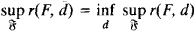# Statistical Decision Theory

## Statistical Decision Theory

an area of mathematical statistics and game theory that permits a number of diverse problems to be treated in a common manner. Such problems include the statistical testing of hypotheses, the obtaining of statistical estimators for parameters and of confidence limits for the estimators, and the design of experiments.

Statistical decision theory is based on the assumption that the probability distribution F of an observed random variable XF belongs to some prior given set ℑ The principal task of statistical decision theory consists of finding the best decision function, or strategy, d = d(x) permitting a judgment to be made as to the true, but unknown, distribution F on the basis of the results of the observations x of X. In order to compare the merits of various decision functions, the loss function W[F, d(x)] is introduced. It represents the loss produced in adopting the decision d(x) (from a specified set D) when the true distribution is F.

It would be natural to regard the decision function d* = d*(x) as best if the average risk r(F, d*) = MFW[F, d(X)] (MF represents averaging over the distribution F) does not exceed r(F, d) for any F £ ℑ and any decision function d = d(x). In most problems, however, such a uniformly best decision function does not exist. For this reason, minimax and Bayes solutions are of great interest in statistical decision theory. The decision function d̄ = d̄(x) is said to be minimax ifThe decision function d̄ = d̄(x) is said to be a Bayes solution (relative to a given prior distribution π in ℑ) if for all decision functions d

R(π, ̄) ≤ R(π, d)

where

R(π, d) = ʃ r(F,d)π(d,F)

A close relation exists between the minimax and Bayes solutions: under extremely broad assumptions regarding the data of the problem, a minimax solution is the Bayes solution relative to the least favorable prior distribution π.

### REFERENCES

Wald, A. “Statisticheskie reshaiushchie funktsii.” In the collection Pozitsionnye igry. Moscow, 1967. (Translated from English.)
Lehmann, E. Proverka statisticheskikh gipotez. Moscow, 1964. (Translated from English.)

A. N. SHIRIAEV

References in periodicals archive ?
Statistical decision theory and Bayesian analysis second edition.
A sample of paper topics includes measurement and research on stage machinery noise in theater, balance and vibration analysis on an in-line five cylinder engine, stability design of cutting current based on fuzzy control theory, skin color detection using the statistical decision theory, improved design on guardrail used for frequent accident occurring highway section, work principle and application of linear motion unit, teaching in technological institutes, risk decision-making system in manufacture enterprise management, preliminary discussion on training mode of excellence engineer in application oriented university, and study on multimedia education system of technological college.
Part I covers basic ideas of matrix theory and statistical decision theory, while Part II examines the estimators, their derivations, and the relationships between estimators from Bayesian and non-Bayesian perspectives.
EP: Statistical decision theory is a game against nature.
The abstract ideas laid out here constitute the building blocks of modern statistical decision theory. (13) No claim of originality is made.
Bernardo and Smith propose some ways of engaging in statistical decision theory when no model is true; they do this in a very special context where the action of the modeler is the choice of a model and the objective of the modeler is the prediction of a future observation.
(31.) See Hurwicz (1951) for a remarkable early suggestion that preferences like those in equation 8 could bridge different variants of statistical decision theory; these types of preferences are discussed in Manski (forthcoming).
If the question is simply whether or not to automate, a straightforward design procedure is available by applying standard statistical decision theory to the failure-response task.
(This association is not surprising, given that signal detection theory can be treated as a special form of statistical decision theory with additional parametric assumptions.) [[beta].sub.opt] is the threshold at which, if for larger values one decides S (signal) and for smaller values one decides N (noise), one maximizes expected return (based on given contingent costs, benefits, and prior probabilities of F).
(For example, most economists, and Laband and Taylor, though they quote Salant on the matter, do not know that phrases like "knowledge claim production function" are unclear.) It would be as though economists did not know some obviously relevant tool such as statistical decision theory (actually, they do not).
Take, for instance, David Blackwell, a mathematician who teaches and researches statistical decision theory at the University of California at Berkeley and is the only living Black member of the prestigious National Academy of Sciences.
Zimmerman discusses the kinds of uncertainties faced by policy makers using the terminology of statistical decision theory.

Site: Follow: Share:
Open / Close